In :
import numpy as np
import pandas as pd
import matplotlib.pyplot as plt
import numpy.random as random
from IPython.display import Image
# seaborn is under active development and throws some scary looking warnings
import warnings
# this will allow us to use the code in peace :)
warnings.filterwarnings("ignore")


#### Lecture 17:¶

• Learn how to use the seaborn package to produce beautiful plots

• Learn about kernel density estimates

• Learn appropriate ways of representing different types of data

### seaborn¶

In this lecture, we will learn about seaborn. seaborn is a package with many tools for data visualization. It allows you to make pretty plots. Almost anything in seaborn can be done using matplotlib, but with seaborn's built-in functions you can reduce a lot of matplotlib code down to a single line. seaborn isn't just a pretty face. Its real power is in statistical data analysis. It has a lot of functions built in for visualizing the distribution of your data, for example.

Let's take a look at some of the plots we can make with this package. We can import it using:

In :
import seaborn as sns


### Unusual distributions, kernel density estimates and jointplots¶

In some cases, we have distributions of data that don't look like a simple (e.g., normal) distribution, for example, the data could be bimodal or have skewed shaped distributions (remember the histogram of the elevation data from around the world with two humps).

Let's create some synthetic bimodal data by drawing from two separate normal/lognormal distributions and combine the two into two bimodal data sets. We do this by drawing from random.normal( ) twice for two normal distributions ($x_1,x_2$) and twice from random.lognormal( ) for two lognormal distributions ($y_1,y_2$).

In :
xdata1=random.normal(20,25,5000) # first x draw
xdata2=random.normal(100,25,5000) # second x draw
ydata1=random.lognormal(2,0.1,8000) # first y draw
ydata2=random.lognormal(3,0.1,2000) # second y draw
xdata=np.append(xdata1,xdata2) # combine the two x data sets
ydata=np.append(ydata1,ydata2) # combine the two y data sets


When we plot our xdata as a histogram, we can see that we have a broadly bimodal distribution. For fun, let's also plot the mean of the distribution as a red line.

In :
# make the histogram
plt.hist(xdata,bins=50)
# put on a heavy (linewidth=3) vertical red line at the mean of xdata
plt.axvline(np.mean(xdata),color='red',linewidth=3);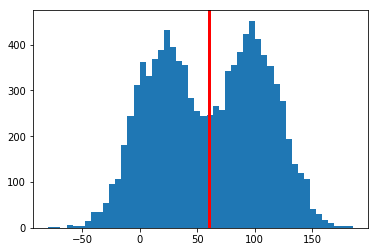We can see that our mean lies right between the twin peaks. Describing this distribution with statistics meant for normal distributions (mean or standard deviation) is just plain wrong.

Another way to represent the distribution of a set of datapoints is known as a kernel density estimate (kde). This places a 'kernel' (an assumed distribution at the data point level - usually a normal distribution) at each data point and then sums up the contributions from all the data points. Kernal density estimates avoid the awkwardness of choice of bin size associated with histograms, for example. (We just picked 50 in the plot above - why 50?).

Here are some data represented on a bar plot in the lefthand plot. And on the right, we illustrate the idea behind kernal density estimates. The black lines are the locations of individual datapoints and the red dashed lines are the kernels at each point. The heavy blue line is the kernel density estimate (the sum of all the red dashed lines).

In :
Image('Figures/KDE.png')

Out: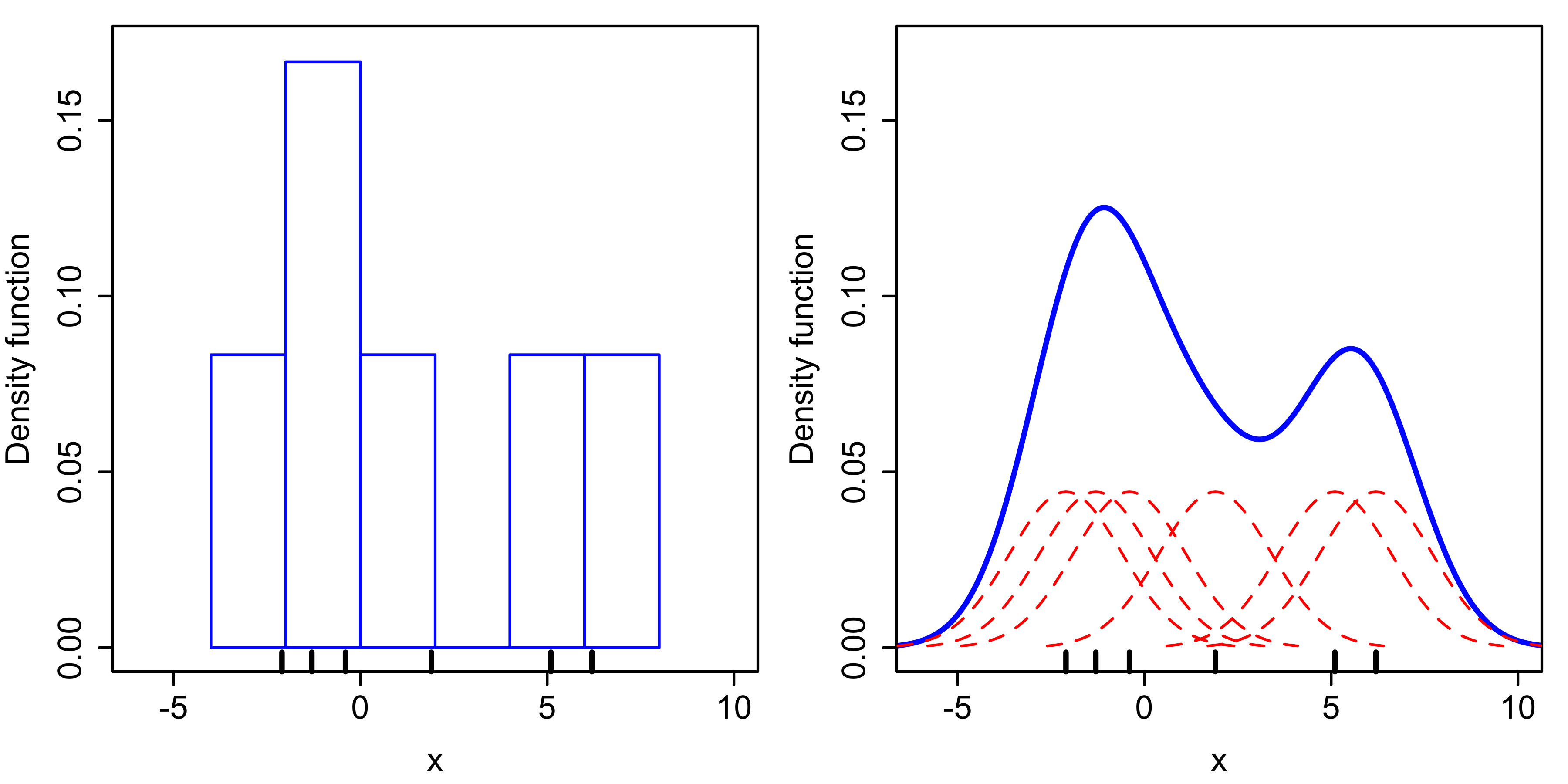Happily, we can plot kernel density estimates using the sns.kdeplot( ) function. The shade argument allows us to shade the area underneath the curve. By the way, in matplotlib, the same thing can be achieved using the function plt.fill_between.

In :
sns.kdeplot(xdata,shade=True);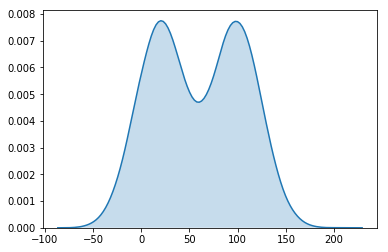Well that was fairly painless! We can also plot the kernal density estimate and the histogram on top of one another using the sns.distplot( ) function.

In :
sns.distplot(xdata);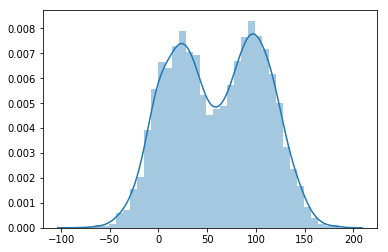As you can see, this is a lot quicker than how we were plotting our distribution in the lecture on statistics!

With our $ydata$ we can see that we also have a bimodal distribution, but there are far fewer data points in the wider mode (we only used 2000 of our 10000 points for this mode).

In :
sns.distplot(ydata);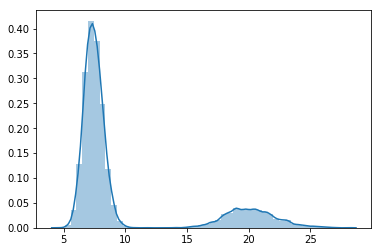What if our data had both $x$ and $y$ components. For example, measurements of length and width from a set of shark teeth with two species in it. How would we visualize it? Let's just try plotting the $x$ data on the x axis and $y$ data on the y axis as dots on a regular matplotlib plot.

In :
plt.plot(xdata,ydata,'.');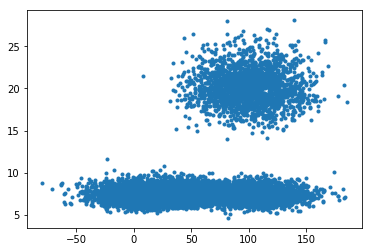It's much harder to see the bimodal distribution in the $x$ data in this case, and we can't really see that there are so much fewer data points in the $y$ data mode than the more tightly clustered one. Also, this is not a plot you would expect from length and width dimensions of teeth from a single species - wouldn't that be a linear plot?

When we have a lot of datapoints, this type of plot gives us no easy way to estimate data density. Fortunately, seaborn includes a cool plot called 'sns.jointplot( ). This combines the histograms and the scatter plot into a single graph.

In :
sns.jointplot(xdata,ydata);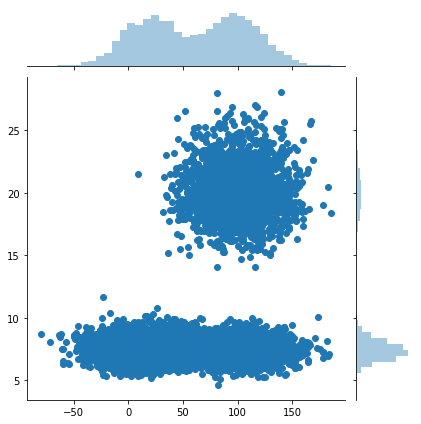Jointplot can also do kernel density estimates! The kind argument on this plot gives us many other options plotting data as well:

In :
help(sns.jointplot)

Help on function jointplot in module seaborn.axisgrid:

jointplot(x, y, data=None, kind='scatter', stat_func=None, color=None, height=6, ratio=5, space=0.2, dropna=True, xlim=None, ylim=None, joint_kws=None, marginal_kws=None, annot_kws=None, **kwargs)
Draw a plot of two variables with bivariate and univariate graphs.

This function provides a convenient interface to the :class:JointGrid
class, with several canned plot kinds. This is intended to be a fairly
lightweight wrapper; if you need more flexibility, you should use
:class:JointGrid directly.

Parameters
----------
x, y : strings or vectors
Data or names of variables in data.
data : DataFrame, optional
DataFrame when x and y are variable names.
kind : { "scatter" | "reg" | "resid" | "kde" | "hex" }, optional
Kind of plot to draw.
stat_func : callable or None, optional
*Deprecated*
color : matplotlib color, optional
Color used for the plot elements.
height : numeric, optional
Size of the figure (it will be square).
ratio : numeric, optional
Ratio of joint axes height to marginal axes height.
space : numeric, optional
Space between the joint and marginal axes
dropna : bool, optional
If True, remove observations that are missing from x and y.
{x, y}lim : two-tuples, optional
Axis limits to set before plotting.
{joint, marginal, annot}_kws : dicts, optional
Additional keyword arguments for the plot components.
kwargs : key, value pairings
Additional keyword arguments are passed to the function used to
draw the plot on the joint Axes, superseding items in the
joint_kws dictionary.

Returns
-------
grid : :class:JointGrid
:class:JointGrid object with the plot on it.

--------
JointGrid : The Grid class used for drawing this plot. Use it directly if
you need more flexibility.

Examples
--------

Draw a scatterplot with marginal histograms:

.. plot::
:context: close-figs

>>> import numpy as np, pandas as pd; np.random.seed(0)
>>> import seaborn as sns; sns.set(style="white", color_codes=True)
>>> g = sns.jointplot(x="total_bill", y="tip", data=tips)

Add regression and kernel density fits:

.. plot::
:context: close-figs

>>> g = sns.jointplot("total_bill", "tip", data=tips, kind="reg")

Replace the scatterplot with a joint histogram using hexagonal bins:

.. plot::
:context: close-figs

>>> g = sns.jointplot("total_bill", "tip", data=tips, kind="hex")

Replace the scatterplots and histograms with density estimates and align
the marginal Axes tightly with the joint Axes:

.. plot::
:context: close-figs

>>> g = sns.jointplot("sepal_width", "petal_length", data=iris,
...                   kind="kde", space=0, color="g")

Draw a scatterplot, then add a joint density estimate:

.. plot::
:context: close-figs

>>> g = (sns.jointplot("sepal_length", "sepal_width",
...                    data=iris, color="k")
...         .plot_joint(sns.kdeplot, zorder=0, n_levels=6))

Pass vectors in directly without using Pandas, then name the axes:

.. plot::
:context: close-figs

>>> x, y = np.random.randn(2, 300)
>>> g = (sns.jointplot(x, y, kind="hex")
...         .set_axis_labels("x", "y"))

Draw a smaller figure with more space devoted to the marginal plots:

.. plot::
:context: close-figs

>>> g = sns.jointplot("total_bill", "tip", data=tips,
...                   height=5, ratio=3, color="g")

Pass keyword arguments down to the underlying plots:

.. plot::
:context: close-figs

>>> g = sns.jointplot("petal_length", "sepal_length", data=iris,
...                   marginal_kws=dict(bins=15, rug=True),
...                   annot_kws=dict(stat="r"),
...                   s=40, edgecolor="w", linewidth=1)



Let's try the kind=kde option. (Warning, doing a 2d kde plot like this is a lot of work so this cell might take a while to run).

In :
sns.jointplot(xdata,ydata,kind='kde');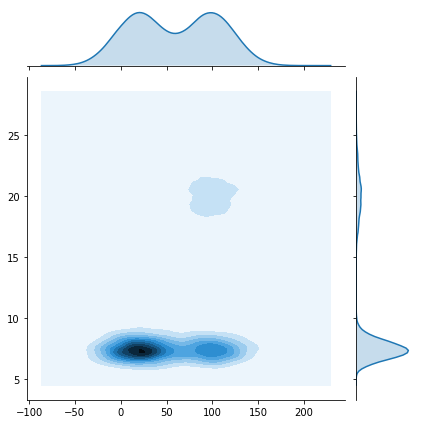Another type of plot that looks nice is the hexbin plot (kind of like a hexagonal 2d histogram)

In :
sns.jointplot(xdata,ydata,kind='hexbin');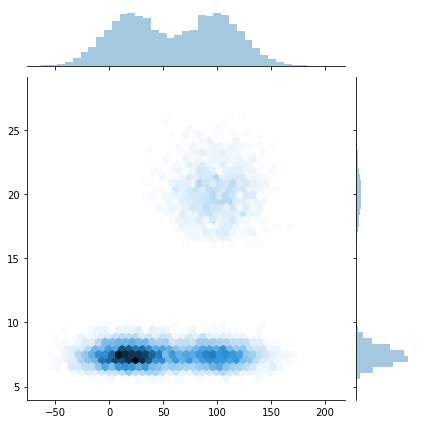### Multi dimensional data and pairplots¶

What if you have data with more than two dimensions? A good example of this is with isotopic data from Ocean Island Basalts. Isotopic systems are used to "finger-print" different sources of melt in the mantle. It is used to characterize what is deep in the Earth using what gets brought up to form the ocean islands. By now there are data available for many different isotopic systems. Here we take a look at a small sample of what is in the GeoRoc database (http://georoc.mpch-mainz.gwdg.de/georoc/) for ocean island basalts.

In :
MantleArray=pd.read_csv('Datasets/GeoRoc/MantleArray_OIB.csv')

Out:
CITATION EPSILON_HF EPSILON_ND HF176_HF177 LAND/SEA (SAMPLING) LATITUDE (MAX.) LATITUDE (MIN.) LOCATION LOCATION COMMENT LONGITUDE (MAX.) ... PB208_PB204 ROCK NAME ROCK TYPE SAMPLE NAME SAMPLING TECHNIQUE SR87_SR86 TECTONIC SETTING TYPE OF MATERIAL UNIQUE_ID Year
0  STILLE P. (1986) 14.534010 7.607708 0.283196 SUBAERIAL 19.83 19.83 HAWAIIAN ISLANDS NaN -155.42 ... 38.017 ANKARAMITE VOLCANIC ROCK samp. 79MK1 OUTCROP 0.70347 OCEAN ISLAND WHOLE ROCK 107-79MK1 1986
1  STILLE P. (1986) 10.538041 6.378770 0.283083 SUBAERIAL 22.00 22.00 HAWAIIAN ISLANDS NAPALI MEMBER -159.50 ... 37.803 THOLEIITE VOLCANIC ROCK samp. KAU-1 OUTCROP 0.70384 OCEAN ISLAND WHOLE ROCK 5-KAU-1 1986
2  STILLE P. (1986) 11.033117 6.281235 0.283097 SUBAERIAL 22.00 22.00 HAWAIIAN ISLANDS NAPALI MEMBER -159.50 ... 37.962 THOLEIITE VOLCANIC ROCK samp. 1D872-2 OUTCROP 0.70364 OCEAN ISLAND WHOLE ROCK NaN 1986
3  STILLE P. (1986) 12.164719 5.696027 0.283129 SUBAERIAL 21.15 21.15 HAWAIIAN ISLANDS NaN -156.97 ... 37.751 THOLEIITE VOLCANIC ROCK samp. 71WMOL-1 OUTCROP 0.70378 OCEAN ISLAND WHOLE ROCK 782-71WMOL-1 1986
4  STILLE P. (1986) 15.135173 5.891097 0.283213 SUBAERIAL 21.16 21.16 HAWAIIAN ISLANDS NaN -157.23 ... 37.754 THOLEIITE VOLCANIC ROCK samp. 71WMOL-3 OUTCROP 0.70376 OCEAN ISLAND WHOLE ROCK 783-71WMOL-3 1986

5 rows × 24 columns

There are lots of different isotope ratios available. In this lecture, we focus on four different isotopic ratios: $^{87}$Sr/$^{86}$Sr , $^{206}$Pb/$^{204}$Pb , $\varepsilon$ Nd and $\varepsilon$ Hf. (The ratios with an $\varepsilon$ are the isotope ratios relative to a standard value in parts per 10000, this allows you to see the variation better as it is normally very small).

So, how do you plot multi-dimensional data? Our new plotting buddy seaborn has a function for multi-dimensional data known as the sns.pairplot( ). This makes a plot that takes data with many dimensions and plots each possible two dimensional combination against one another in a grid where each of the rows and columns represents a dimension. When a particular dimension is plotted against itself, however, it gives the kernal density estimates for the different data types (set by the keyword argument, hue). In this case we will be using $LOCATION$ to group the data).

In our isotopic example, the top row plots $\varepsilon$ Nd against all of the other isotope ratios in turn with the first plot on the left being the kernal density plot for each location with each in a different color.

Let's see it in action (warning, cell may take a while to run because of the large number of locations):

In :
sns.pairplot(MantleArray,\
vars=['EPSILON_ND','SR87_SR86','PB206_PB204','EPSILON_HF'],\
hue='LOCATION');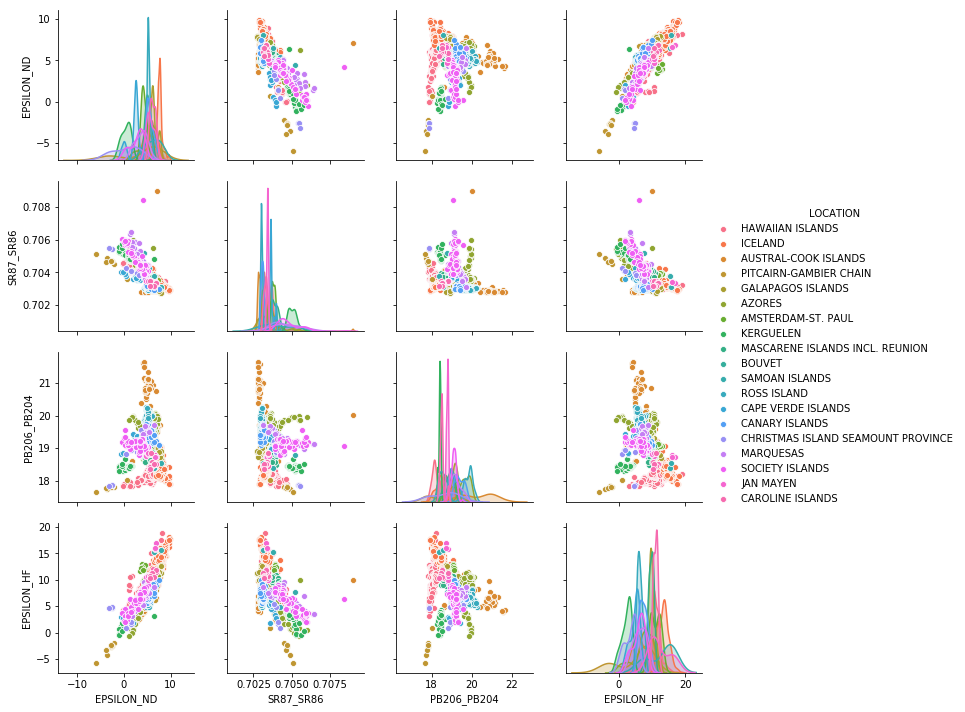sns.pairplot( ) gives us an interesting way of seeing trends in multidimensional space. Some ocean islands have quite different isotopic compositions from others! It's important to note that some projections of these data (e.g. $^{206}$Pb/$^{204}$Pb vs $\varepsilon$ Nd) show this effect more prominently whereas others, like $\varepsilon$ Nd vs $\varepsilon$ Hf, are more subtle. In this plot (second from the bottom on the left), the Austral-Cook Islands are all the way on the top to the right, and Pitcairn is way at the bottom to the left.

It might be surprising to some of you who know a little geophysics that there is heterogeneity between different ocean islands. You might have thought that the mantle is very well mixed by convection. Many geochemists believe that the source regions for the plumes that form these basalts have different compositions because subduction brings down crustal material with different compositions to the base of the mantle, and this material then upwells in plumes that form the ocean islands. According to that hypothesis, the variation in isotopic ratios we see here comes from mixing between these recycled crustal end members and a mantle composition.

We will look further at this dataset when we learn how to plot things in three dimensions and also when we group the data into 'end-members' using a bit of machine learning.

### Box and whisker, violin plots and swarm plots¶

The kernal density estimate plots in the element versus itself plots in the sns.pairplot( ) plot example above plot all of the isotopic data on one plot. This doesn't really allow us to appreciate fully the variability at a given location. There are two additional plots that might give us a better idea of that: the 'box and whisker' plot (very popular, but I hate them) and the 'violin' plots (love these!).

seaborn has functions (actually classes) for both of these, sns.boxplot( ) and sns.violinplot( ). In these plots, we can also place the data (and not just the distributions) and can use the handy sns.swarmplot( ) to spread out the data along the x-axis so that we can see them better. We'll go through each of these tricks in the following.

First, the boxplot:

In :
help(sns.boxplot)

Help on function boxplot in module seaborn.categorical:

boxplot(x=None, y=None, hue=None, data=None, order=None, hue_order=None, orient=None, color=None, palette=None, saturation=0.75, width=0.8, dodge=True, fliersize=5, linewidth=None, whis=1.5, notch=False, ax=None, **kwargs)
Draw a box plot to show distributions with respect to categories.

A box plot (or box-and-whisker plot) shows the distribution of quantitative
data in a way that facilitates comparisons between variables or across
levels of a categorical variable. The box shows the quartiles of the
dataset while the whiskers extend to show the rest of the distribution,
except for points that are determined to be "outliers" using a method
that is a function of the inter-quartile range.

Input data can be passed in a variety of formats, including:

- Vectors of data represented as lists, numpy arrays, or pandas Series
objects passed directly to the x, y, and/or hue parameters.
- A "long-form" DataFrame, in which case the x, y, and hue
variables will determine how the data are plotted.
- A "wide-form" DataFrame, such that each numeric column will be plotted.
- An array or list of vectors.

In most cases, it is possible to use numpy or Python objects, but pandas
objects are preferable because the associated names will be used to
annotate the axes. Additionally, you can use Categorical types for the
grouping variables to control the order of plot elements.

This function always treats one of the variables as categorical and
draws data at ordinal positions (0, 1, ... n) on the relevant axis, even
when the data has a numeric or date type.

See the :ref:tutorial <categorical_tutorial> for more information.

Parameters
----------
x, y, hue : names of variables in data or vector data, optional
Inputs for plotting long-form data. See examples for interpretation.
data : DataFrame, array, or list of arrays, optional
Dataset for plotting. If x and y are absent, this is
interpreted as wide-form. Otherwise it is expected to be long-form.
order, hue_order : lists of strings, optional
Order to plot the categorical levels in, otherwise the levels are
inferred from the data objects.
orient : "v" | "h", optional
Orientation of the plot (vertical or horizontal). This is usually
inferred from the dtype of the input variables, but can be used to
specify when the "categorical" variable is a numeric or when plotting
wide-form data.
color : matplotlib color, optional
Color for all of the elements, or seed for a gradient palette.
palette : palette name, list, or dict, optional
Colors to use for the different levels of the hue variable. Should
be something that can be interpreted by :func:color_palette, or a
dictionary mapping hue levels to matplotlib colors.
saturation : float, optional
Proportion of the original saturation to draw colors at. Large patches
often look better with slightly desaturated colors, but set this to
1 if you want the plot colors to perfectly match the input color
spec.
width : float, optional
Width of a full element when not using hue nesting, or width of all the
elements for one level of the major grouping variable.
dodge : bool, optional
When hue nesting is used, whether elements should be shifted along the
categorical axis.
fliersize : float, optional
Size of the markers used to indicate outlier observations.
linewidth : float, optional
Width of the gray lines that frame the plot elements.
whis : float, optional
Proportion of the IQR past the low and high quartiles to extend the
plot whiskers. Points outside this range will be identified as
outliers.
notch : boolean, optional
Whether to "notch" the box to indicate a confidence interval for the
median. There are several other parameters that can control how the
notches are drawn; see the plt.boxplot help for more information
on them.
ax : matplotlib Axes, optional
Axes object to draw the plot onto, otherwise uses the current Axes.
kwargs : key, value mappings
Other keyword arguments are passed through to plt.boxplot at draw
time.

Returns
-------
ax : matplotlib Axes
Returns the Axes object with the plot drawn onto it.

--------
violinplot : A combination of boxplot and kernel density estimation.
stripplot : A scatterplot where one variable is categorical. Can be used
in conjunction with other plots to show each observation.
swarmplot : A categorical scatterplot where the points do not overlap. Can
be used with other plots to show each observation.

Examples
--------

Draw a single horizontal boxplot:

.. plot::
:context: close-figs

>>> import seaborn as sns
>>> sns.set(style="whitegrid")
>>> ax = sns.boxplot(x=tips["total_bill"])

Draw a vertical boxplot grouped by a categorical variable:

.. plot::
:context: close-figs

>>> ax = sns.boxplot(x="day", y="total_bill", data=tips)

Draw a boxplot with nested grouping by two categorical variables:

.. plot::
:context: close-figs

>>> ax = sns.boxplot(x="day", y="total_bill", hue="smoker",
...                  data=tips, palette="Set3")

Draw a boxplot with nested grouping when some bins are empty:

.. plot::
:context: close-figs

>>> ax = sns.boxplot(x="day", y="total_bill", hue="time",
...                  data=tips, linewidth=2.5)

Control box order by passing an explicit order:

.. plot::
:context: close-figs

>>> ax = sns.boxplot(x="time", y="tip", data=tips,
...                  order=["Dinner", "Lunch"])

Draw a boxplot for each numeric variable in a DataFrame:

.. plot::
:context: close-figs

>>> ax = sns.boxplot(data=iris, orient="h", palette="Set2")

Use hue without changing box position or width:

.. plot::
:context: close-figs

>>> tips["weekend"] = tips["day"].isin(["Sat", "Sun"])
>>> ax = sns.boxplot(x="day", y="total_bill", hue="weekend",
...                  data=tips, dodge=False)

Use :func:swarmplot to show the datapoints on top of the boxes:

.. plot::
:context: close-figs

>>> ax = sns.boxplot(x="day", y="total_bill", data=tips)
>>> ax = sns.swarmplot(x="day", y="total_bill", data=tips, color=".25")

Use :func:catplot to combine a :func:pointplot and a
:class:FacetGrid. This allows grouping within additional categorical
variables. Using :func:catplot is safer than using :class:FacetGrid
directly, as it ensures synchronization of variable order across facets:

.. plot::
:context: close-figs

>>> g = sns.catplot(x="sex", y="total_bill",
...                 hue="smoker", col="time",
...                 data=tips, kind="box",
...                 height=4, aspect=.7);



Let's look at the $\epsilon$ Hf data as a function of location (similar to the lower right hand corner plot in the pairplot example above:

In :
box=sns.boxplot(data=MantleArray,x='LOCATION',y='EPSILON_HF')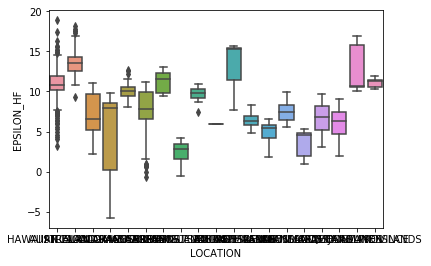Oh my. Pretty plot, but the x axis tick labels overlap so badly we can't even see them. To rotate them, we use the figure object as we have done before. Instead of fig, we will call the figure object box in the following. This will let us use plt.setp( ) to set properties (such as the x tick labels) on our plot object, box.

In :
help(plt.setp)

Help on function setp in module matplotlib.pyplot:

setp(*args, **kwargs)
Set a property on an artist object.

matplotlib supports the use of :func:setp ("set property") and
:func:getp to set and get object properties, as well as to do
introspection on the object.  For example, to set the linestyle of a
line to be dashed, you can do::

>>> line, = plot([1,2,3])
>>> setp(line, linestyle='--')

If you want to know the valid types of arguments, you can provide
the name of the property you want to set without a value::

>>> setp(line, 'linestyle')
linestyle: [ '-' | '--' | '-.' | ':' | 'steps' | 'None' ]

If you want to see all the properties that can be set, and their
possible values, you can do::

>>> setp(line)
... long output listing omitted

You may specify another output file to setp if sys.stdout is not
acceptable for some reason using the file keyword-only argument::

>>> with fopen('output.log') as f:
>>>     setp(line, file=f)

:func:setp operates on a single instance or a iterable of
instances. If you are in query mode introspecting the possible
values, only the first instance in the sequence is used. When
actually setting values, all the instances will be set.  e.g.,
suppose you have a list of two lines, the following will make both
lines thicker and red::

>>> x = arange(0,1.0,0.01)
>>> y1 = sin(2*pi*x)
>>> y2 = sin(4*pi*x)
>>> lines = plot(x, y1, x, y2)
>>> setp(lines, linewidth=2, color='r')

:func:setp works with the MATLAB style string/value pairs or
with python kwargs.  For example, the following are equivalent::

>>> setp(lines, 'linewidth', 2, 'color', 'r')  # MATLAB style
>>> setp(lines, linewidth=2, color='r')        # python style


In :
box=sns.boxplot(data=MantleArray,x='LOCATION',y='EPSILON_HF')
plt.setp(box.get_xticklabels(), rotation=90);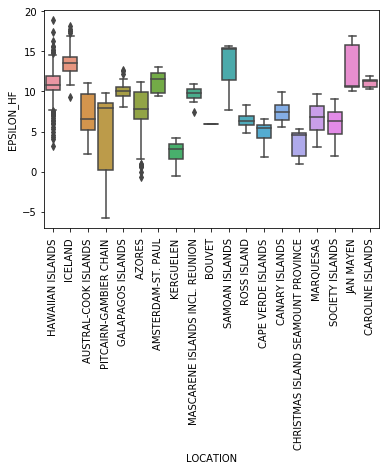The x axis labels are better, but the plot still doesn't look great. The black diamonds are known as 'fliers' and could represent "outliers".

As we have some pretty large "outliers" in this dataset, we need to either limit the range on the y axis or by making the plot larger. These fliers are annoying (although they represent something that maybe we shouldn't be ignoring...). It is possible to exclude them from the box and whisker plot, using the argument fliersize=0.

You need to be aware that this can be a problematic practice as you are effectively hiding data from the viewer. Personally, I wouldn't do it... But lot's of folks do, so here is how:

In :
plt.figure(figsize=(10,8)) # make the plot bigger
box=sns.boxplot(data=MantleArray,x='LOCATION',y='EPSILON_HF',fliersize=0)
plt.setp(box.get_xticklabels(), rotation=90);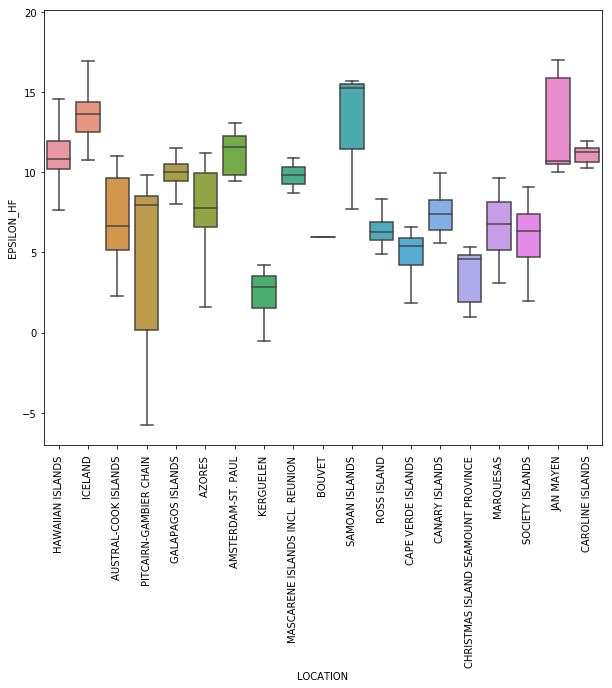See! This looks much better. But looks can be deceiving!

Alternatively, we can put the data back on, but use a white dot with a black edge, and spread them out so we can see them.
In order to see the data points that overlap one another, seaborn has an option to plot the data points in a cloud using the function sns.swarmplot( ). Here we do both of these (and also make the points white with a black edge). The argument alpha=0.5 is used here to make the points semi transparent. (alpha sets 'opacity' so alpha=1 is fully opaque and alpha=0, points are invisible).

In :
plt.figure(figsize=(20,10))
box=sns.boxplot(data=MantleArray,x='LOCATION',y='EPSILON_HF',fliersize=0)
plt.setp(box.get_xticklabels(), rotation=90);
sns.swarmplot(data=MantleArray,x='LOCATION',y='EPSILON_HF',color='white',edgecolor='black',linewidth=1,alpha=0.5);